Translate the power of knowledge into action. Open Free* Demat Account

# The error ratio

4.6y = mx + c

Here, y is the dependent variable, while x is the independent variable.

Now, when you are actually trading a pair of stocks, how do you determine which one to take as the dependent one and which as the independent one? After all, you can run the regression both ways. Let’s do that first and then go about figuring out which of the two stocks - TCS and Infosys - gets to be x and which gets to be y.

### Regression function with the TCS stock as the dependent variable

If you’ll recall from the previous chapter, this was the setup with which we ran the original regression function. So, here’s the screenshot of the input and the output.

Running the function: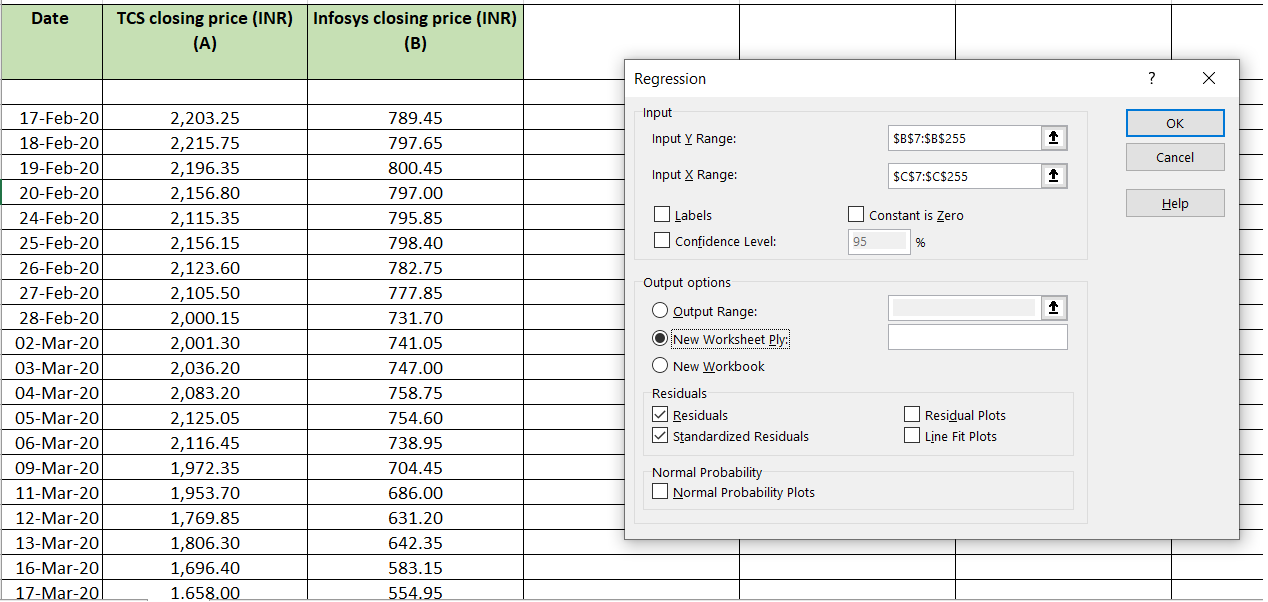Output: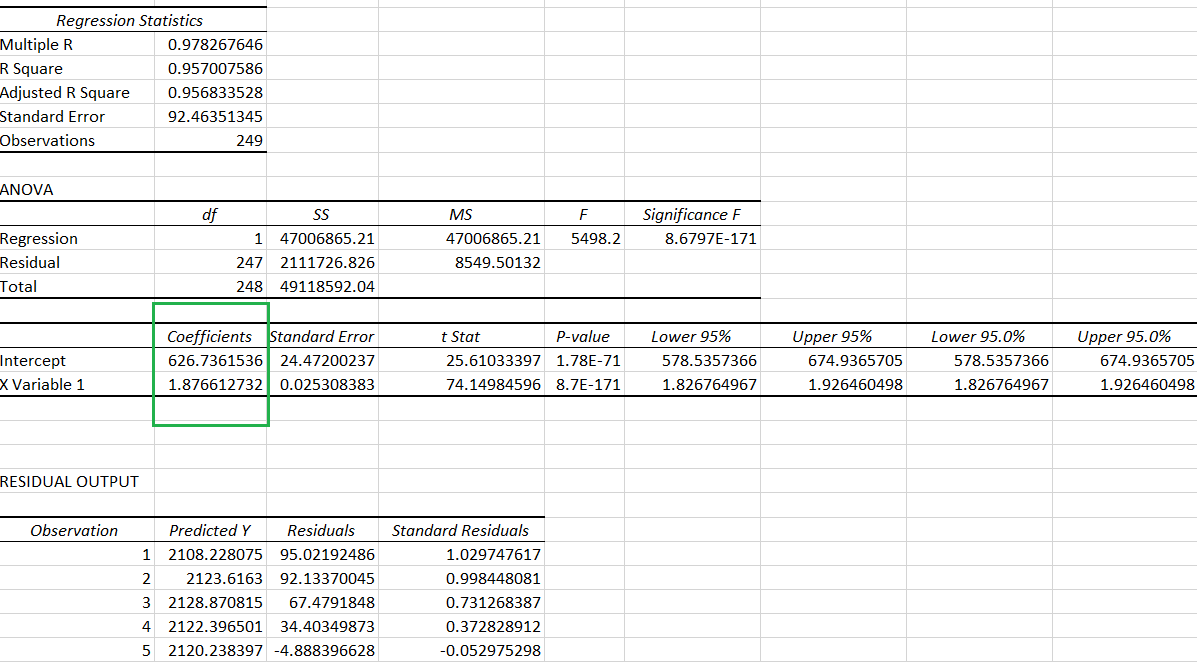So, the equation we’re looking at is: y = 1.8766x + 626.74

Or, TCS share price = (1.8766 times Infosys share price) + 626.74

### Regression function with the Infosys stock as the dependent variable

Now, let’s reverse the x and y and set up the regression run with Infosys as the dependent stock. Here’s the screenshot of the input and the output.

Running the function: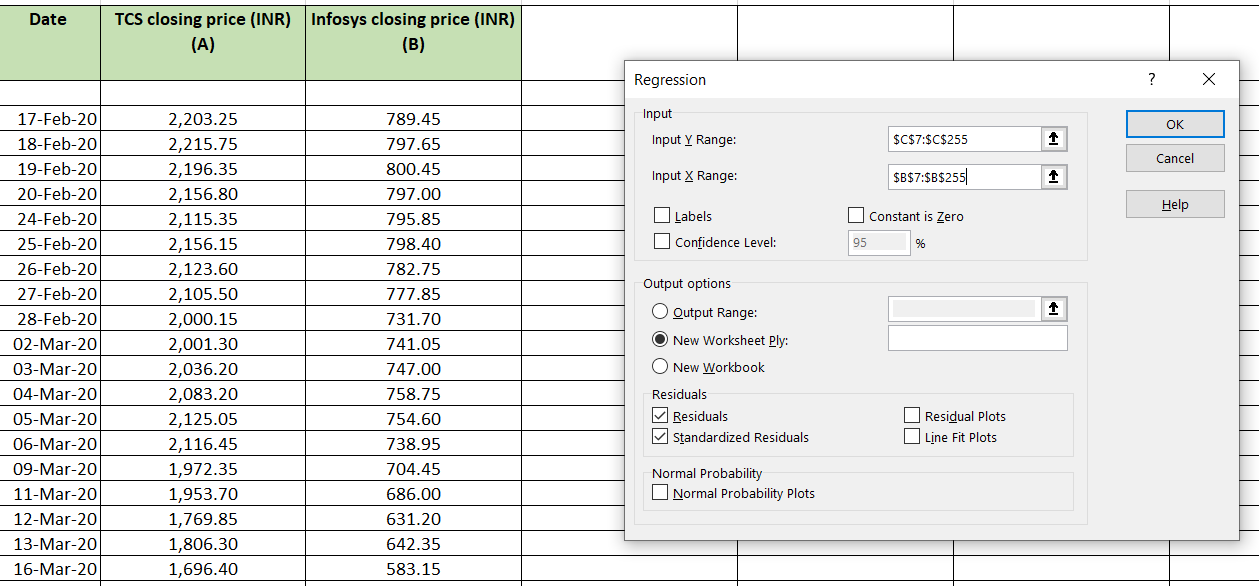Output: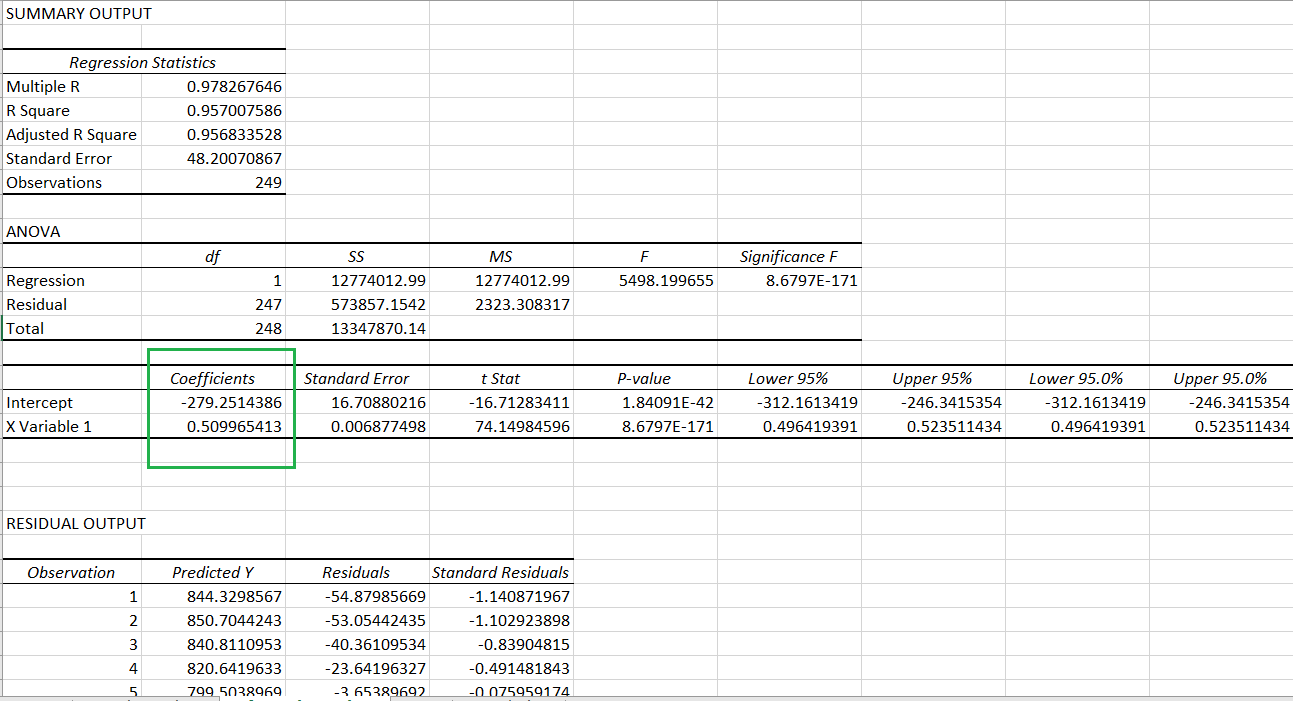So, the equation we’re looking at is: y = 0.5099x - 279.25

Or, Infosys share price = (0.5099 times TCS share price) - 279.25

### The error ratio

As we saw in the previous chapter, the straight line equations obtained from the regression run are not 100% true for all the data points. That’s why we have residuals in the first place. But, as with all calculations involving huge numbers of observations, there’s bound to be an error in either setup.

And logic dictates that we should assign x and y to the two shares based on whichever arrangement comes with the least amount of error. That’s where the error ratio comes in handy.

Check out the formula for this here.

 Error ratio = Standard error of intercept ÷ Standard error

The standard error in the denominator is essentially the standard deviation of the residuals for each data pair. You can calculate this to cross-check the accuracy, if you wish to.

And, don’t worry about encountering more numbers, because you’ll find both the standard error of intercept as well as the standard error in the output data. Let’s see where this information is located.

### Error ratio with the TCS stock as the dependent variable

This is the output for the regression function with the TCS stock as the dependent variable.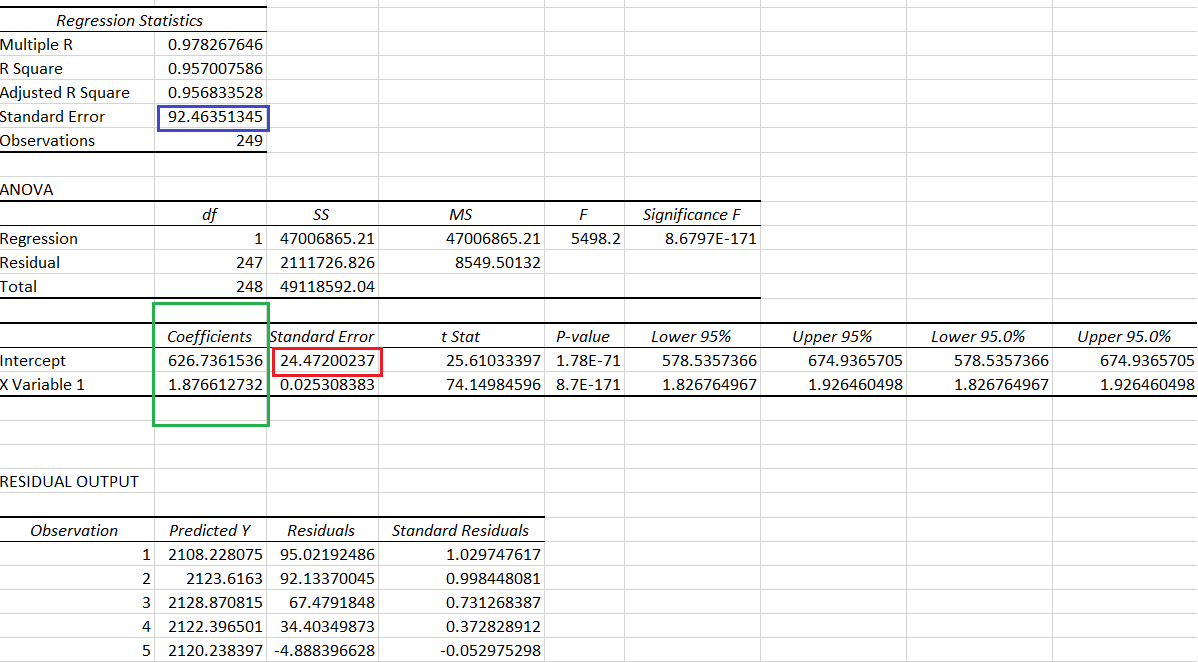Note the following things:

• The standard error of intercept has been marked in a red box. The value, as you can see, is 24.47
• The standard error has been marked in a blue box. It is 92.46

### Error ratio with the Infosys stock as the dependent variable

This is the output for the regression function with the Infosys stock as the dependent variable.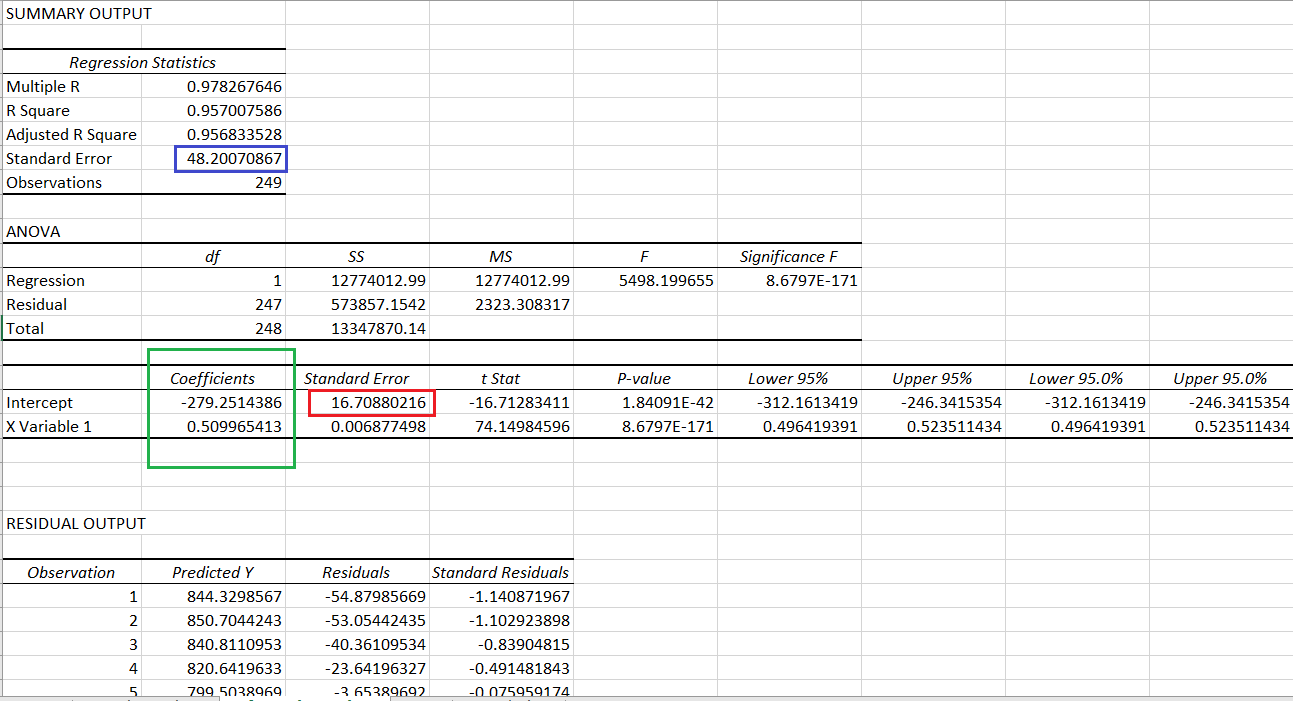Note the following things:

• The standard error of intercept has been marked in a red box. The value is 16.71
• The standard error has been marked in a blue box. It is 48.2

### Calculating the error ratio in both the arrangements

 Dependent Independent Standard error of intercept (A) Standard error (B) Error ratio (A ÷ B) TCS Infosys 24.47 92.46 0.264 Infosys TCS 16.70 48.20 0.346

#### Wrapping up

As you can see, taking TCS as the dependent variable (y) and Infosys as the independent variable (x) gives the lower error ratio. So, for the sake of analysing the data to identify a trigger for pair trading, this is what we’ll use as x and y:

• Independent variable x: Infosys stock price
• Dependent variable y: TCS stock price

#### A quick recap

• The regression function can be run keeping either variable as dependent and independent.
• The decision to consider one stock price as dependent and the other as independent is based on the error ratio in the two iterations.
• The lower the error ratio, the better.
• The error ratio is calculated as the standard error of intercept ÷ standard error